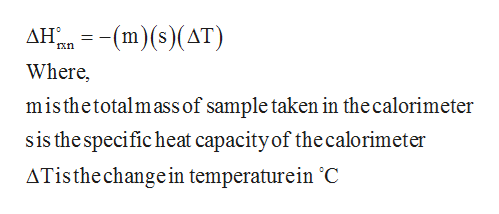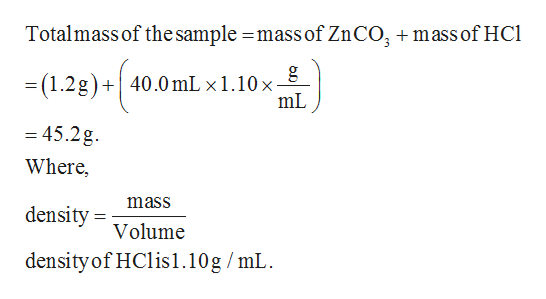# A 1.200 g sample of ZnCO3 (s) dissolved in 40.0 mL of a dilute HCl solution (density = 1.10 g/mL) in a coffee cup calorimeter, producing CO2 (g), liquid water and ZnCl2 (aq).  The temperature of the solution changes from 24.32 oC to 25.49 oC.  The resulting solution has a specific heat of 4.18 J/goC.What would be the # of heat released by the reaction and Is the reaction endothermic?

Question
1 views

A 1.200 g sample of ZnCO3 (s) dissolved in 40.0 mL of a dilute HCl solution (density = 1.10 g/mL) in a coffee cup calorimeter, producing CO2 (g), liquid water and ZnCl2 (aq).  The temperature of the solution changes from 24.32 oC to 25.49 oC.  The resulting solution has a specific heat of 4.18 J/goC.

What would be the # of heat released by the reaction and Is the reaction endothermic?

check_circle

Step 1

The required equation is given below.

Step 2

The heat of the reaction in a calorimeter can be calculated by using the given below formula.help_outlineImage TranscriptioncloseAH2(m)s)(AT) Where, misthetotalmass of sample taken in the calorimeter sis the specific heat capacity of the calorimeter ATisthe changein temperaturein 'C fullscreen
Step 3

The heat of the given reaction in a calorime...help_outlineImage TranscriptioncloseTotalmass of thesample =mass of ZnCO, mass of HCl gg = (1.2g)+40.0 mL x 1.10 x - mL = 45.2g Where, mass density Volume density of HClis1.10g /mL fullscreen

### Want to see the full answer?

See Solution

#### Want to see this answer and more?

Solutions are written by subject experts who are available 24/7. Questions are typically answered within 1 hour.*

See Solution
*Response times may vary by subject and question.
Tagged in

### Physical Chemistry